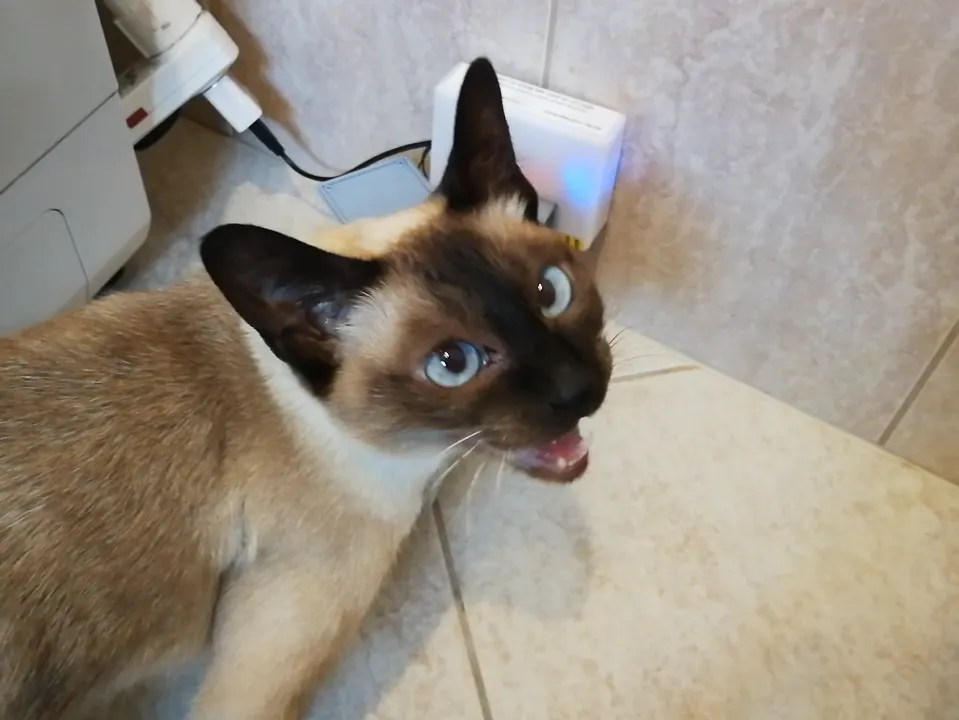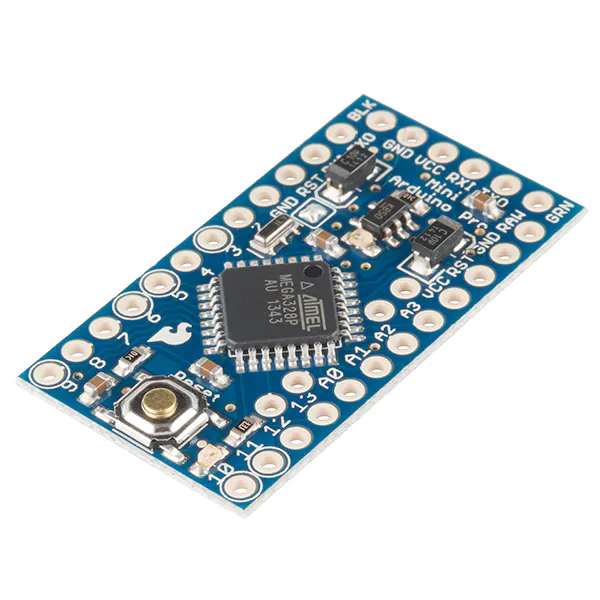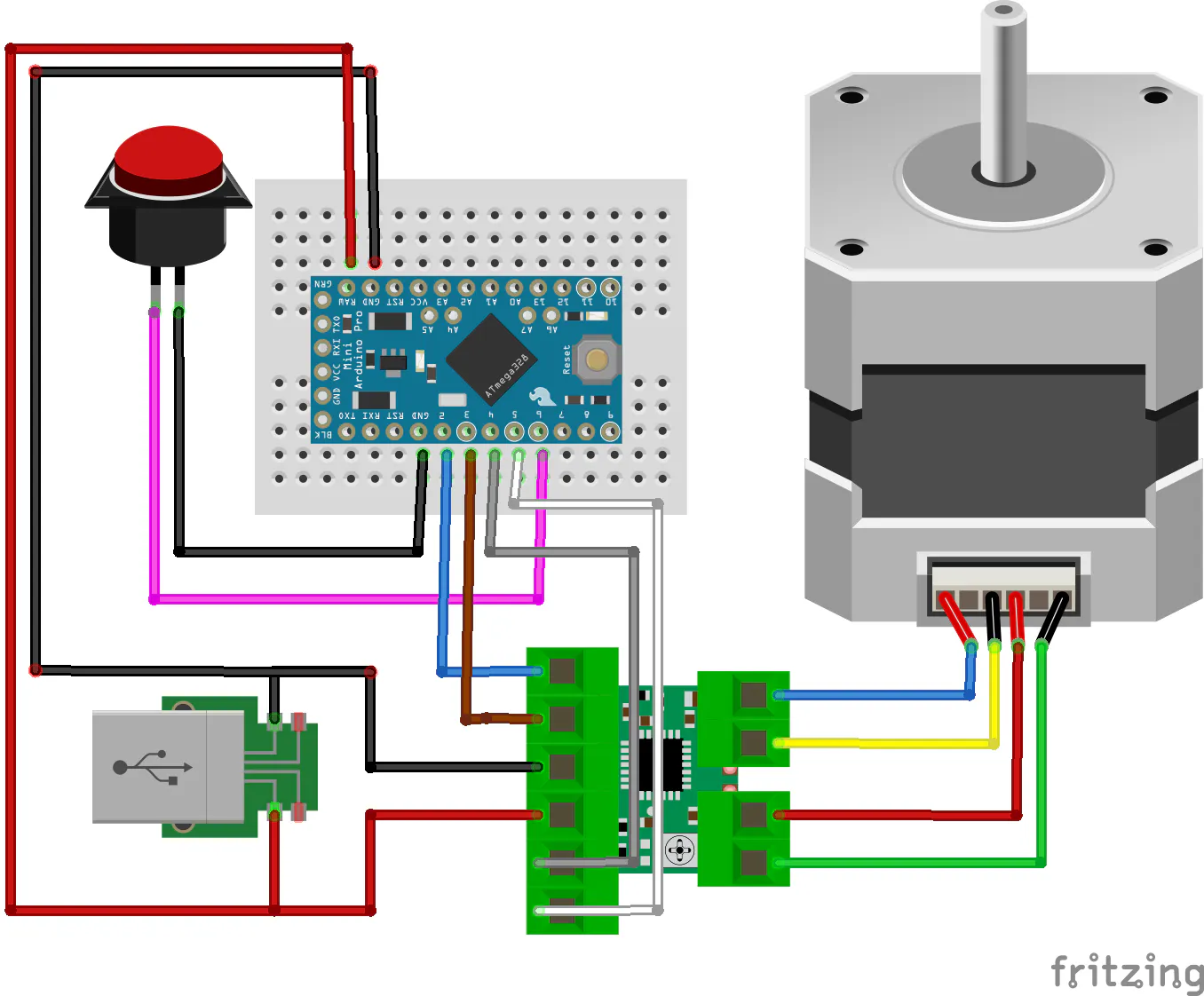# DIY Automatic Cat Feeder

This is a homemade automatic cat feeder instruction. Simple to use and easy to build, no special tools or materials are needed.

IntermediateFull instructions provided3 hours25,157## Things used in this project

### Hardware components

 Mechanical Outlet Timer
×1
 Stepper Motor
×1
 Stepper Motor Driver L9110S
×1
 Linear Shaft Support SHF8
×1
 USB Wall Charger
×1SparkFun Arduino Pro Mini 328 - 5V/16MHz
×1
 USB Male Connectors to DIP Adapter
×1
 USB Extension Cable
×1
 Brackets corner brace
×1

## Schematics

### Automatic cat feeder schematic

First, I did the cabling of the electronics. I used only twice my soldering iron to connect the power cables to the USB adapter PCB. Before connecting to the 230V network I recommend to use a laboratory bench power supply with current control and current measurement. if you cabled something wrongly, this checking could save you any damage on the adapter or at another component. The whole consumption should be less than 1 Amper at 5 Volt.
Secondly, I assembled the corner braces, then modified the box and fixed to the structure of the machine.## Code

### Automatic_cat_feeder arduino code

C/C++
Arduino firmware source code. I uploaded the code to the Arduino pro mini board with the help of Arduino IDE software (https://www.arduino.cc/en/Main/Software), and FTDI adapter and a Mini-B USB cable. Here is a video on, how to do this process: https://www.youtube.com/watch?v=78HCgaYsA70
The code is simple. In the setup, it will turn the stepper than in the main loop it waits until the button is pressed then it will move once again.
```// Automatic cat feeder made by: J. Rundhall

//Original code for steper from :Sketch by R. Jordan Kreindler, written October 2016, to rotate

float RPM;

boolean isButtonpressed = false;

unsigned long timee;

// Pin assignments

int buttonPIN = 6;

int aPin = 4; //IN1: coil a one end

int bPin = 3; //IN2: coil b one end

int aPrimePin = 5; //IN3: coil aPrime other end of coil a

int bPrimePin = 2; //IN4: coil bPrime other end of coil b

int one = aPin;

int two = bPin;

int three = aPrimePin;

int four = bPrimePin;

int degrees = 0;

//int delay1 = 20; // The delay between each step in milliseconds
int delay1 = 5; // The delay between each step in milliseconds

//int delay2 = 50; // The delay after each full revolution, in milliseconds
int delay2 = 200; // The delay after each full revolution, in milliseconds

int count = 0; // The number of steps

int numberOfRotations = 1; // The number of times the rotor has

// turned 360 degrees.

void setup() {

// Set all pins as output to send output signals from the Arduino

// UNO to the coil windings of the stator

Serial.begin(9600);     // opens serial port, sets data rate to 9600 bps

pinMode(6, INPUT_PULLUP);  //Button

pinMode(aPin, OUTPUT);

pinMode(bPin, OUTPUT);

pinMode(aPrimePin, OUTPUT);

pinMode(bPrimePin, OUTPUT);

Serial.println(" Clockwise");

digitalWrite(aPin, LOW);

digitalWrite(bPin, LOW);

digitalWrite(aPrimePin, LOW);

digitalWrite(bPrimePin, LOW);

for(int ii=0;ii<20;ii++)
doTurn();

}

void loop() {

//read the pushbutton value into a variable
// Keep in mind the pull-up means the pushbutton's logic is inverted. It goes
// HIGH when it's open, and LOW when it's pressed. Turn on pin 13 when the
// button's pressed, and off when it's not:
if (sensorVal == LOW) {
isButtonpressed = true;
} else {
if(isButtonpressed)
{
isButtonpressed = false;
doTurn();
digitalWrite(aPin, LOW);

digitalWrite(bPin, LOW);

digitalWrite(aPrimePin, LOW);

digitalWrite(bPrimePin, LOW);
}

}
}

void doTurn()
{

// Send current to

// 1. The aPin

// 2. The aPin, and the bPin

// 3. The bPin

// 4. Then to the bPin and the aPrimePin

// 5. Then to the aPrimePin

// 6. Then to the aPrimePin and the bPrime Pin

// 7. Then to the bPrimePin

// 8. Then the bPrimePin and the aPin.

// Thus producing steps using the half step method

// 1. Set the aPin High

digitalWrite(aPin, HIGH);

digitalWrite(bPin, LOW);

digitalWrite(aPrimePin, LOW);

digitalWrite(bPrimePin, LOW);

// Allow some delay between energizing the coils to allow

// the stepper rotor time to respond.

delay(delay1); // So, delay1

// 2. Energize aPin and bPin to HIGH

digitalWrite(aPin, HIGH);

digitalWrite(bPin, HIGH);

digitalWrite(aPrimePin, LOW);

digitalWrite(bPrimePin, LOW);

// Allow some delay between energizing the coils to allow

// the stepper rotor time to respond.

delay(delay1); // So, delay1 milliseconds

// 3. Set the bPin to High

digitalWrite(aPin, LOW);

digitalWrite(bPin, HIGH);

digitalWrite(aPrimePin, LOW);

digitalWrite(bPrimePin, LOW);

// Allow some delay between energizing the coils to allow

// the stepper rotor time to respond.

delay(delay1); // So, delay1 milliseconds

// 4. Set the bPin and the aPrimePin to HIGH

digitalWrite(aPin, LOW);

digitalWrite(bPin, HIGH);

digitalWrite(aPrimePin, HIGH);

digitalWrite(bPrimePin, LOW);

// Allow some delay between energizing the coils to allow

// the stepper rotor time to respond.

delay(delay1); // So, delay1 milliseconds

// 5. Set the aPrime Pin to high

digitalWrite(aPin, LOW);

digitalWrite(bPin, LOW);

digitalWrite(aPrimePin, HIGH);

digitalWrite(bPrimePin, LOW);

// Allow some delay between energizing the coils to allow

// the stepper rotor time to respond.

delay(delay1); // So, delay1 milliseconds

// 6. Set the aPrimePin and the bPrime Pin to HIGH

digitalWrite(aPin, LOW);

digitalWrite(bPin, LOW);

digitalWrite(aPrimePin, HIGH);

digitalWrite(bPrimePin, HIGH);

// Allow some delay between energizing the coils to allow

// the stepper rotor time to respond.

delay(delay1); // So, delay1 milliseconds

// 7. Set the bPrimePin to HIGH

digitalWrite(aPin, LOW);

digitalWrite(bPin, LOW);

digitalWrite(aPrimePin, LOW);

digitalWrite(bPrimePin, HIGH);

// Allow some delay between energizing the coils to allow

// the stepper rotor time to respond.

delay(delay1); // So, delay1 milliseconds

// 8. Set the bPrimePin and the aPin to HIGH

digitalWrite(aPin, HIGH);

digitalWrite(bPin, LOW);

digitalWrite(aPrimePin, LOW);

digitalWrite(bPrimePin, HIGH);

// Allow some delay between energizing the coils to allow

// the stepper rotor time to respond.

delay(delay1); // So, delay1 milliseconds

count = count + 8;

degrees = (360.0 * (count / 400.0));

if ((numberOfRotations % 2) == 1) { // Check if number of rotations is even

Serial.println(" Clockwise ");

Serial.println(degrees); // Print angular position in degrees

}

else { // If numberOfRotations is odd number

Serial.println(" Anti-Clockwise ");

degrees = 360 - degrees;

Serial.print(" -"); // Print a minus sign

Serial.println(degrees); // Print angular position in degrees

}

if (count == 160) { // A full revolution of the stepper

numberOfRotations = ++numberOfRotations;

timee = millis();

RPM = timee / numberOfRotations; // Average time of a rotation

RPM = (60000.00 / RPM); // Number of rotations per minute

if (numberOfRotations >= 10) {

Serial.print("RPM:");

Serial.println(round(RPM)); //Print RPM as integer

}

delay(delay2); // delay2/1000 second(s) after each full rotation

count = 0; // Reset step counter to zero

//Reversive direction after each turn

if ((numberOfRotations) % 2 == 0) { // Check if number of rotations is even

// if so reverse direction

aPin = four;

bPin = three;

aPrimePin = two;

bPrimePin = one;

}

else { // If number of rotations is an odd number

aPin = one;

bPin = two;

aPrimePin = three;

bPrimePin = four;

}
digitalWrite(aPin, LOW);

digitalWrite(bPin, LOW);

digitalWrite(aPrimePin, LOW);

digitalWrite(bPrimePin, LOW);
}

}
```

## Credits

Thanks to R. Jordan Kreindler.